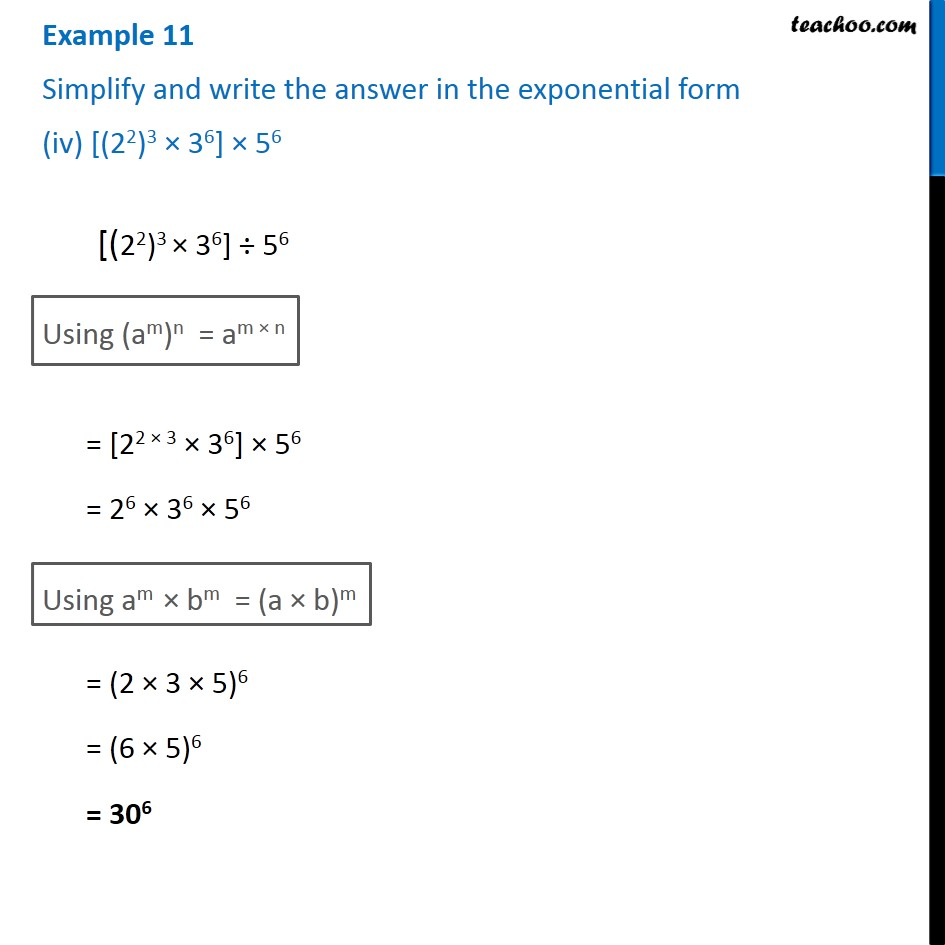1. Chapter 13 Class 7 Exponents and Powers
2. Serial order wise
3. Examples

Transcript

Example 11 Simplify and write the answer in the exponential form (iv) [(22)3 × 36] × 56 [(22)3 × 36] ÷ 56 Using (am)n = am × n = [22 × 3 × 36] × 56 = 26 × 36 × 56 Using am × bm = (a × b)m = (2 × 3 × 5)6 = (6 × 5)6 = 306